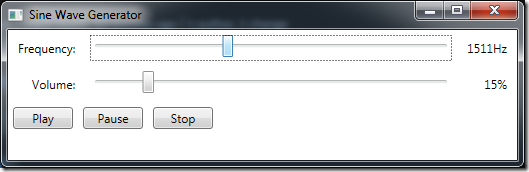Here’s a code sample I’ve been meaning to share on my blog for years. NAudio already has a built-in `SignalGenerator` class which can generate sine waves as well as various other waveforms. But what if you want to implement “portamento” to glide smoothly between frequencies?

One simple way to do this is to make use of a “wavetable”. Basically we store one cycle of a sine wave in a memory buffer, and then to work out what the next sample to be played is, we move forward a certain number of slots in that buffer and read out the value. If we go past the end of the buffer we simply start again.

If we call the current position in the waveform the “phase” and the number of steps in the wavetable we must move forward the “phase step”, then when the target frequency is changed, instead of immediately recalculating a new “phase step”, we slowly change the current phase step until it reaches the desired phase step.

It’s quite a simple technique, and the great thing is that it works for any waveform, so you could quite easily do the same with square or sawtooth waveforms.

Here’s a really basic implementation of this, which allows you to customise the portamento time. I meant for this setting to be in seconds, but I think I’ve got it slightly wrong as when you set it to 1.0 it seems to take longer than a second to reach the target frequency.

``````class SineWaveProvider : ISampleProvider
{
private float[] waveTable;
private double phase;
private double currentPhaseStep;
private double targetPhaseStep;
private double frequency;
private double phaseStepDelta;
private bool seekFreq;

public SineWaveProvider(int sampleRate = 44100)
{
WaveFormat = WaveFormat.CreateIeeeFloatWaveFormat(sampleRate, 1);
waveTable = new float[sampleRate];
for (int index = 0; index < sampleRate; ++index)
waveTable[index] = (float)Math.Sin(2 * Math.PI * (double)index / sampleRate);
// For sawtooth instead of sine: waveTable[index] = (float)index / sampleRate;
Frequency = 1000f;
Volume = 0.25f;
PortamentoTime = 0.2; // thought this was in seconds, but glide seems to take a bit longer
}

public double PortamentoTime { get; set; }

public double Frequency
{
get
{
return frequency;
}
set
{
frequency = value;
seekFreq = true;
}
}

public float Volume { get; set; }

public WaveFormat WaveFormat { get; private set; }

public int Read(float[] buffer, int offset, int count)
{
if (seekFreq) // process frequency change only once per call to Read
{
targetPhaseStep = waveTable.Length * (frequency / WaveFormat.SampleRate);

phaseStepDelta = (targetPhaseStep - currentPhaseStep) / (WaveFormat.SampleRate * PortamentoTime);
seekFreq = false;
}
var vol = Volume; // process volume change only once per call to Read
for (int n = 0; n < count; ++n)
{
int waveTableIndex = (int)phase % waveTable.Length;
buffer[n + offset] = this.waveTable[waveTableIndex] * vol;
phase += currentPhaseStep;
if (this.phase > (double)this.waveTable.Length)
this.phase -= (double)this.waveTable.Length;
if (currentPhaseStep != targetPhaseStep)
{
currentPhaseStep += phaseStepDelta;
if (phaseStepDelta > 0.0 && currentPhaseStep > targetPhaseStep)
currentPhaseStep = targetPhaseStep;
else if (phaseStepDelta < 0.0 && currentPhaseStep < targetPhaseStep)
currentPhaseStep = targetPhaseStep;
}
}
return count;
}
}
``````

I’ve packaged this up into a very simple WPF application available on GitHub for you to try out. One word of warning, sine waves can be ear piercing so start off with the volume set very low before trying this out.Please note, my wavetable code is extremely rudimentary. If you want to go into the science behind doing this properly, make sure to check out Nigel Redmon’s excellent wavetable series at Ear Level Engineering. This involves creating multiple wavetables to cover different frequency ranges and using linear interpolation (I just truncate the phase to the nearest array entry).

Want to get up to speed with the the fundamentals principles of digital audio and how to got about writing audio applications with NAudio? Be sure to check out my Pluralsight courses, Digital Audio Fundamentals, and Audio Programming with NAudio.